×

Search anything:

# Hashing: Complete Guide

#### Algorithms HashingGet this book -> Problems on Array: For Interviews and Competitive Programming

# Contents

1. Introduction
2. Hash Table
3. Hash Function
4. Methods to calculate Hashing Function
1. Division Method
2. Folding Method
3. Mid-Square Method
4. Digit Analysis
5. Collision
6. Techniques to resolve Collision
1.Linear probing Method
3.Double Hashing Technique
7. Conclusion

# Introduction

In hashing, we convert key to another value.It is a searching technique. In linear search the time complexity is O(n),in binary search it is O(log(n)) but in hashing it will be constant.We make use of a hash function and a hash table.

# Hash Table

In hash table the data stored will have unique index value.The time to access becomes very low if we know the index.

# Hash Function

It is used to implement the hash table.It returns the index.

# Methods to calculate Hashing Function

## 1. Division Method

Here the key is divided with a number and we will take the remainder.Mostly we divide it with prime number.The hash function is:f(x)=x%m.Therefore the index can vary from 0 to m-1

## 2. Folding Method

Divides the key into some parts and add each parts.
Example: 45 23 7 = 45+23+7 = 75 which will be the index.This is called shift folding.

### Folding at Boundary

The key is split and the alternative ones are reversed and added.
Example: 24 35 6 = 24+35+6 = 65 which will be the index.

## 3. Mid-Square Method

In this method we will take the hashing function as the square of the key and take the middle value of it.
Example:Let k=9087,k^2=82573569.The middle value is 73 which will be the index.

## 4. Digit Analysis

In this method we already know the key values.We analyse the key and take the digits which appaear frequently at fixed positions and the reverse it.Let key=87654 ,In this we analysed that the 2nd and 4th digit appear frequently that is 7 and 5.Therefore the index is 57.

# Collision

Assume the hash function as h(k)=k%m and m=10 where m is the size of the hash table.The index value will vary from 0 to 9. Let the key be 6.Then h(6)=6%10=6.
Therefore 6 will be stored at index 6.Now let k=26.h(26)=26%10=6 but index 6 already has a value.This is called Collision.

# Techniques to resolve Collision

Several techniques are used to minimize the collision.

It has Chaining method.Assume the given key values are 3,2,9,6,11,13,7,12.We have to store these values to the hash table and the size of hash table is m=10.The hash function is h(k)=2k+3.We have to use Division method and Closed Addressing to store the values.In division method the funtion is k%m.Now let us find the osition of the keys.
The first key is 3.So as we are given a has function and are told to use division method we use the transformation given by 2k+3%10.For 3,2k+3%10 = (2*3)+3%10=9.So we
will store 3 at index 9.

key value
3 * (23)+3%10=9*

The hash table will look like: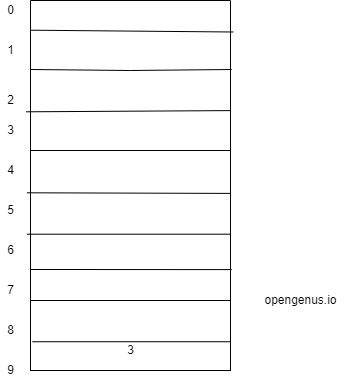Now we will repeat this for values 2,9 and 6.

key value
3 * (23)+3%10=9
2 * (22)+3%10=7
9 * (29)+3%10=1
6 * (26)+3%10=5

The hash table will look like: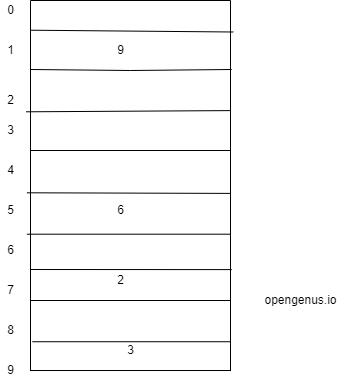Now when we calculate for 11 ,(211)+3%10=5*,but index 5 already contains the value 6.So it is a collision .To resolve it we use chaining method as it is told in the question.In this method at index 5,we will form a linked list and store 6 there.A chain will be formed.The hash table will look like: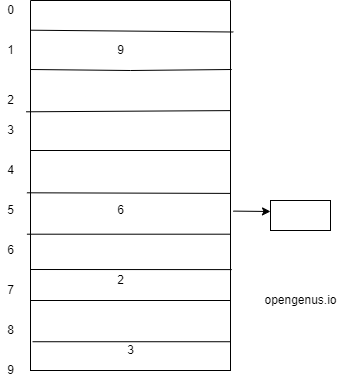Now lets calculate for the value 13.Here (213)+3%10=9*,but index 9 is already filled with 3.So it is a collision.

key value
3 * (23)+3%10=9
2 * (22)+3%10=7
9 * (29)+3%10=1
6 * (26)+3%10=5
11 (211)+3%10=5*
13 (213)+3%10=9*

Therefore at index 9,we will form a linked list and store 13 there.A chain will be formed.The hash table will look like: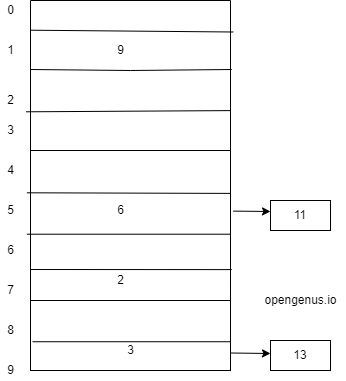For the next value 7.Here (27)+3%10=7*,but index 7 is already filled with 2.So it is a collision.

key value
3 * (23)+3%10=9
2 * (22)+3%10=7
9 * (29)+3%10=1
6 * (26)+3%10=5
11 (211)+3%10=5*
13 (213)+3%10=9*
7 (27)+3%10=7*

Therefore at index 7,we will form a linked list and store 7 there.A chain will be formed.The hash table will look like: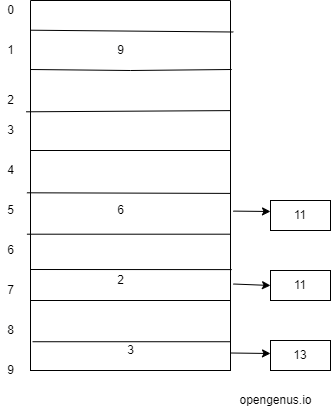For the next value 12.Here (212)+3%10=7*,but index 7 is already filled with 2,then 7 in the chain.So it is a collision.

key value
3 * (23)+3%10=9
2 * (22)+3%10=7
9 * (29)+3%10=1
6 * (26)+3%10=5
11 (211)+3%10=5*
13 (213)+3%10=9*
7 (27)+3%10=7*
12 (212)+3%10=7*

Therefore at index 7,after 7 we will add one more link to store 12. The hash table will look like: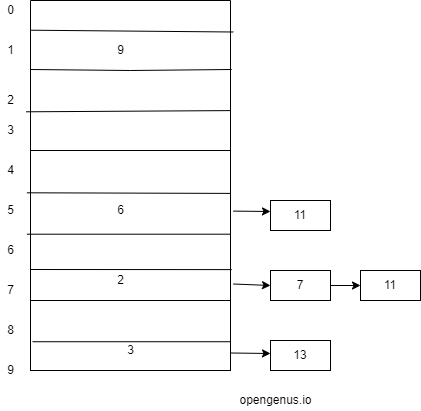Thus all the key values are processed and stored in the table using chaining method.

## 1. Linear probing Method

Assume the given key values are 3,2,9,6,11,13,7,12.We have to store these values to the hash table and the size of hash table is m=10.The hash function is h(k)=2k+3.We have to use Division method and open Addressing to store the values.In division method the funtion is k%m.In open Addressing we will use Linear probing by default.
So for values 3,2,9,6 we will insert it into the table.

key value
3 * (23)+3%10=9
2 * (22)+3%10=7
9 * (29)+3%10=1
6 * (26)+3%10=5

The hash table will look like:Now when we calculate for 11 ,(211)+3%10=5*,but index 5 already contains the value 6.So it is a collision .To resolve it we use linear probing method.Probe means searching.It will search the next free place.The next place here is 6,it is free so we store 11 at index 6.Here the number of probes=2 as we have visited index 5 and 6.

key value probes
3 * (23)+3%10=9 1
2 * (22)+3%10=7 1
9 * (29)+3%10=1 1
6 * (26)+3%10=5 1
11 (211)+3%10=5* 2

Now for 13,(213)+3%10=9*,but index 9 is already filled with 3.So it is a collision.We will search the next free place.The next place here is 0,it is free so we store 11 at index 0.Here the number of probes=2 as we have visited index 9 and 0.

key value probes
3 * (23)+3%10=9 1
2 * (22)+3%10=7 1
9 * (29)+3%10=1 1
6 * (26)+3%10=5 1
11 (211)+3%10=5* 2
13 (213)+3%10=9* 2

The hash table will look like: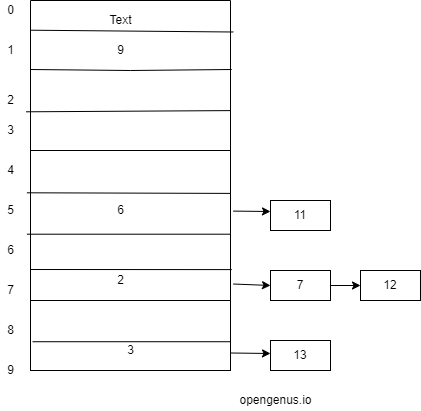Similarly we do for the rest of the keys.

key value probes
3 * (23)+3%10=9 1
2 * (22)+3%10=7 1
9 * (29)+3%10=1 1
6 * (26)+3%10=5 1
11 (211)+3%10=5* 2
13 (213)+3%10=9* 2
7 (27)+3%10=7* 2
12 (212)+3%10=7* 6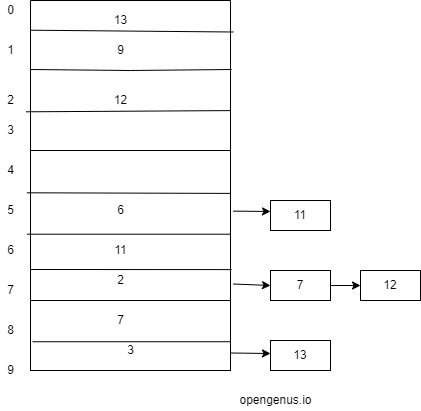The order of the elements are:13,9,12,-,-,6,11,2,7,3.

When collision occurs to find the next free slot we will use a quadratic polynomial.
Assume the given key values are 3,2,9,6,11,13,7,12.We have to store these values to the hash table and the size of hash table is m=10.The hash function is h(k)=2k+3.We have to use Division method and Quadratic probing to store the values.In division method the funtion is k%m
For values 3,2,9,6 we will insert it into the table.

key value
3 * (23)+3%10=9
2 * (22)+3%10=7
9 * (29)+3%10=1
6 * (26)+3%10=5

The hash table will look like:Now when we calculate for 11 ,(211)+3%10=5*,but index 5 already contains the value 6.So it is a collision .To resolve it we use quadratic probing method.To find the next free location we add i^2 to the current index where i begins with 0 and is then incremented at each step.So (5+0^2)%10=5 which already contains an element.Now increment i,i=1, then (5+1^2)%10=6.Index 6 is free so we will store 6 there.Number of probes=2 as we have visited 5 then 6.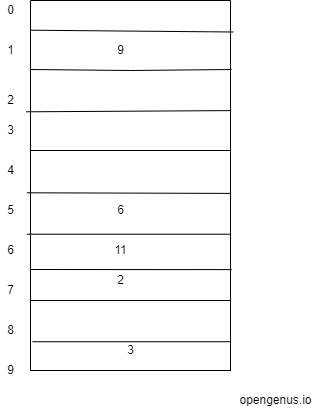For the next value 13,(213)+3%10=9*,but index 9 is already filled with 3.So it is a collision.We will search the next free place.(9+0^2)%10=9 it is not free so increment i,(9+1^2)%10=0,it is free so store 13 at index 0.Similarly we store 7 at index 8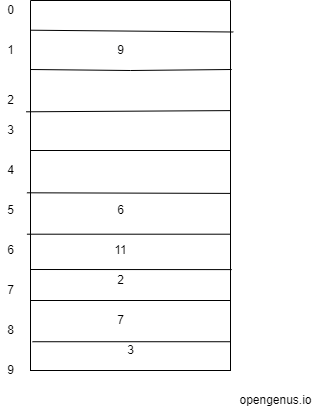Now for 12,(212)+3%10=7*.Collision occurs.So we do quadratic probing.(7+0^2)%10=7 which is already filled.(7+1^2)%10=8 ,Collision occurs so increment i,(7+2^2)%10=1,
which is already filled.Increment i,(7+3^2)%10=6 Collision occurs so increment i,(7+4^2)%10=3,it is free so store 12 at index 3.Number of probes is 5.In linear probing it was 6.So quadratic probing is better.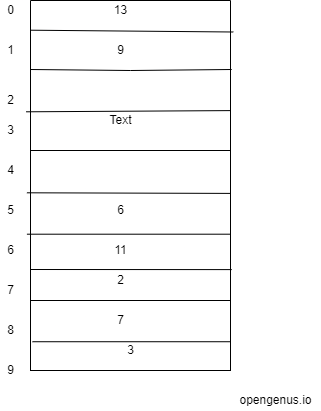The order of the elements is:
13,9,-,12,-,6,11,2,7,3.

## Double Hashing Technique

Insert k at first free place from (u+vi)%m,where u is current index,v=h2(k)%m where m is size of hash table.We apply h2(k) only when there is collision.
Assume the given key values are 3,2,9,6,11,13,7,12.We have to store these values to the hash table and the size of hash table is m=10.The hash function is h(k)=2k+3.We have to use Division method and double hashing technique to store the values.h2(k)=3k+1.
for values 3,2,9,6 we will insert it into the table.

key value v probe
3 * (23)+3%10=9 - 1
2 * (22)+3%10=7 - 1
9 * (29)+3%10=1 - 1
6 * (26)+3%10=5 - 1

The hash table will look like:for 11 ,(211)+3%10=5*,but index 5 already contains the value 6.So it is a collision.Calculating v=(311+1)%10*=4.So calculating (u+vi)%m = (5+40)%10=5.
but it is already filled so i will become 1.
(5+41)%10=9 ,but index 9 is not free.So i=2,(5+42)%10=3*,it is free.so insert 11 at 3.Total number of probes is 3.

key value v probe
3 * (23)+3%10=9 - 1
2 * (22)+3%10=7 - 1
9 * (29)+3%10=1 - 1
6 * (26)+3%10=5 - 1
11 (211)+3%10=5* 4 3

The hash table will look like: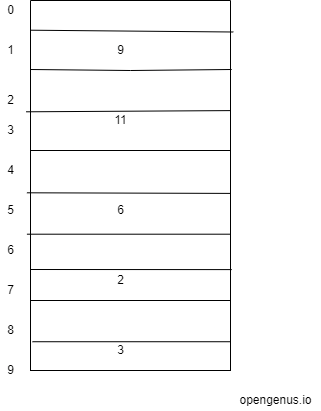Now for 13,(213)+3%10=9*,but index 9 is already filled with 3.So it is a collision.Calculating v=(313+1)%10*=0.So calculating (u+vi)%m = (9+00)%10=9.
As v=0,always we get (u+vi)%m as 9.So it cannot be inserted in the table.Similarly we do for 7 and 12.We cannot insert 7 as when we increment i it will reach greater than m.

key value v probe
3 * (23)+3%10=9 - 1
2 * (22)+3%10=7 - 1
9 * (29)+3%10=1 - 1
6 * (26)+3%10=5 - 1
11 (211)+3%10=5* 4 3
13 (213)+3%10=9* - -
7 (27)+3%10=7* 2 -
12 (212)+3%10=7* 7 2

The hash table will look like: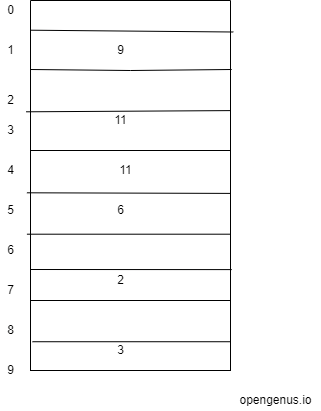The order of the elements is: -,9,-,11,12,6,-,2,-,3.

# Conclusion